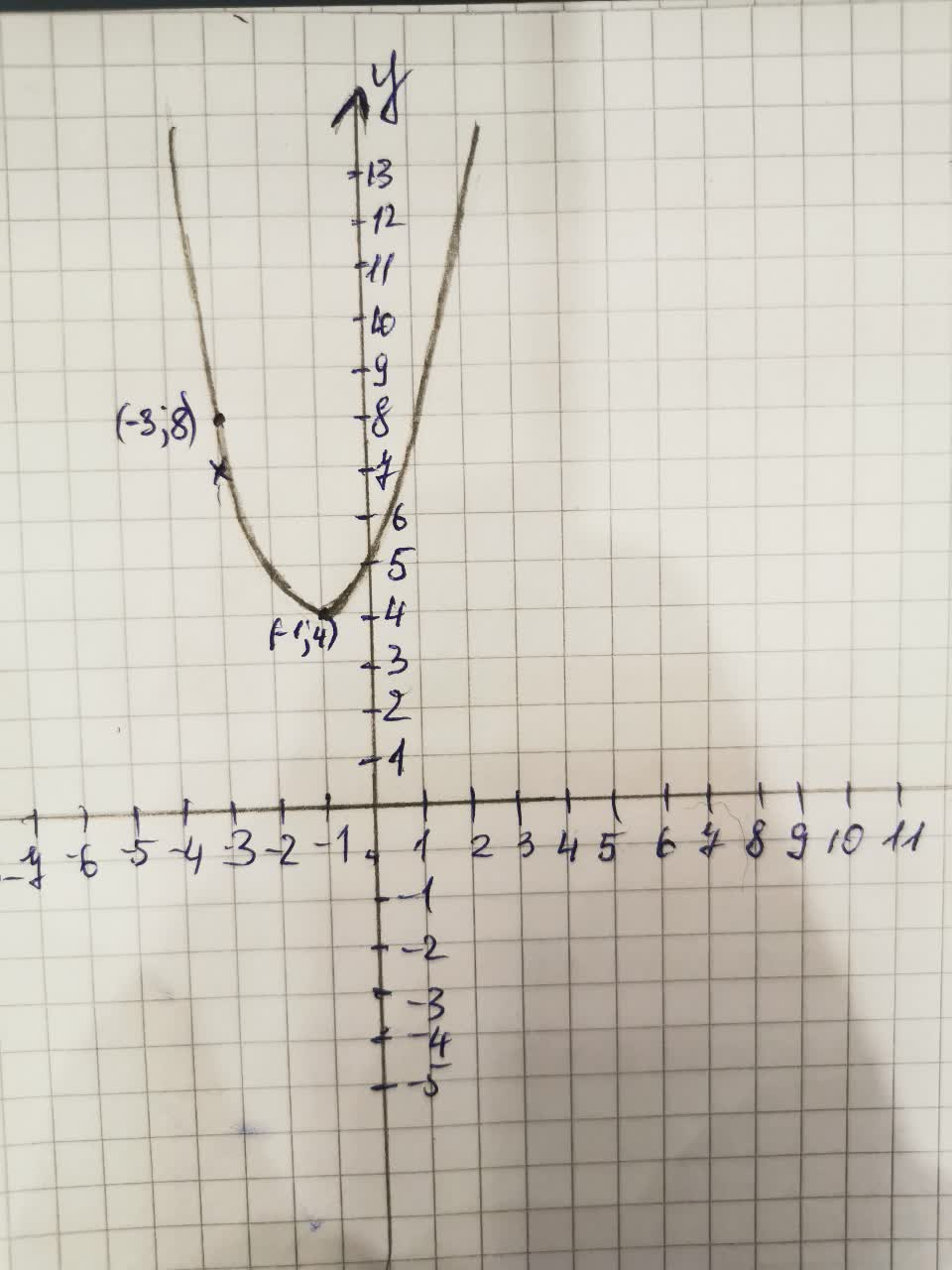# Get help with quadratic functions and equationsRecent questions in Quadratic function and equation
ANSWERED### Differentiate the function: $$\displaystyle{h}{\left({x}\right)}=\frac{{3}}{{4}}{\left({x}^{{5}}–{4}{x}^{{3}}+{6}\right)}^{{8}}$$

ANSWERED### $$\displaystyle{x}^{{2}}+{8}{x}+{15}$$

ANSWERED### Find the equation of the quadratic function whose graph is a parabola containing the points(0.5), (4.41) and (-1.11)

ANSWERED### Which is the equation for the family of quadratic functions with x-intercepts 3 and -2?

ANSWERED### Calculate the product: (x - 7)(x + 8)

ANSWERED### Graph the following equations and explain why they are not graphs of functions of x. |x |+ | y | = 1

ANSWERED### Find the equation of the quadratic function f whose graph is shown belowANSWERED### Solve the quadratic equation by factoring $$25x^2=16$$

ANSWERED### Solve the inequality: $$\displaystyle{5}{x}^{{2}}-{3}{x}{>}{8}$$

ANSWERED### Simplify the following expression: $$\displaystyle\frac{{2}}{{{y}+{3}}}-\frac{{1}}{{{y}^{{2}}+{7}{y}+{12}}}$$

ANSWERED### Find y, if $$\displaystyle{2}{{\cos}^{{2}}{\left({y}\right)}}−{\cos{{\left({y}\right)}}}−{1}={0}$$

ANSWERED### $$\displaystyle{10}{x}^{{2}}-{40}={0}$$

ANSWERED### Find the coordinats if: $$\displaystyle{y}={x}^{{2}}+{3}{x}$$ and y=4x+6

ANSWERED### Show that the function is a linear transformation. Is it also an isomoporphism? T(f(t))=t(f'(t)) from $$\displaystyle{P}_{{{2}}}\to{P}_{{{2}}}$$

ANSWERED### Graph the following quadratic function $$\displaystyle{f{{\left({x}\right)}}}={x}^{{2}}-{4}{x}-{1}$$

ANSWERED### Consider the funstion (x)=$$\displaystyle\sqrt{{{x}-{1}}}+{10}$$ for the domain $$\displaystyle{\left[{1},\infty\right)}$$ Find $$\displaystyle{{f}^{{-{1}}}{\left({x}\right)}}$$, where $$\displaystyle{f}^{{-{1}}}$$ is the inverse of f. Also state the domain of $$\displaystyle{f}^{{-{1}}}$$ in interval notation

ANSWERED### $$\displaystyle{\left({x}+{2}\right)}{\left({x}-\sqrt{{3}}{i}\right)}{\left({x}+\sqrt{{3}}{i}\right)}$$

ANSWERED### $$\displaystyle{2}{x}²+{4}{x}+{1}={0}$$

ANSWERED### Simplify $$\displaystyle{x}^{{2}}−{3}{x}−{4}÷{\left({x}+{2}\right)}$$
ANSWERED